Next: Optimization Up: benches Previous: Polynomial systems

• La page de J-P. Merlet
• J-P. Merlet home page
• La page Présentation de COPRIN
• COPRIN home page
• La page "Présentation" de l'INRIA
• INRIA home page

Subsections

# Non-polynomial systems

## AOL-cosh1

Origin:AOL
3 equations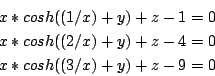Ranges: [-100,100]

Solving method: GradientSolve+HullConsistency+3B
Solutions:: 1 (exact)
Computation time (October 2004):

 DELL D400 (1.7GHz) 5.96s

## Non algebraic: AOL-legentil

3 equations

10/3*cos(x)/sin(x)^2+4*(1+tan(x)^2)/cos(y)+z*(-50/3*sin(y)*cos(x)/(sin(x)^2*(3.5-5*sin(y)))-10/3*cos(x)/sin(x)^2-4*(1+tan(x)^2)/cos(y))=0

4*tan(x)*sin(y)/cos(y)^2+z*(50/3*cos(y)/(sin(x)*(3.5-5*sin(y)))+250/3*sin(y)*cos(y)/(sin(x)*(3.5-5*sin(y))^2)-4*tan(x)*sin(y)/cos(y)^2)=0

50/3*sin(y)/(sin(x)*(3.5-5*sin(y)))+20+10/3/sin(x)-4*tan(x)/cos(y)=0

Ranges: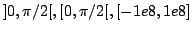for x,y,z
Solving method: GradientSolve+3B+Simp2B
Solutions:: 0
Computation time:
 DELL D620 (1.7GHz), (May 2007): 9mn
Note that this problem may easily be solved by using the Weierstrass substitution to transform the equations into algebraic one and using resultants to obtain an univariate polynomial that leads to all solutions.

## AOL-log1

Origin:AOL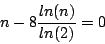Range: [1,1000]

Solving method: GradientSolve
Solutions:: 2 (exact)
Computation time (February 2005):

 Sun Blade 0.06s

## Box3

Origin: 
Let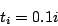and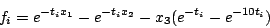for i =1,2,3
Range: [-100,100],[-100,100],[0.1,100]

Solving method: HessianSolve,3B,2B
Solutions:: 1 (exact)
Computation time (April 2003):

 EVO 410C (1.2GHz) 10.4s

## Branin system

Origin: mentioned by Rump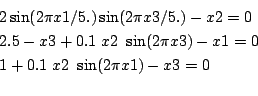Ranges: for all unknowns [-1e8,1e8]

Solving method: GradientSolve,3B,HullConsistency
Solutions:: 1 (exact)
Computation time (September 2006):

 DELL D400 (1.7GHz) 0.01s

## Bratu

Physical meaning: PDE in combustion theory
Origin: unknown

Let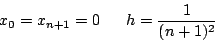and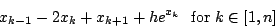with n=15 Unknowns: x,.., xn
Range: [-1e8,20]

Solving method: HessianSolve,HullConsistency
Solutions:: 2 pour n=15, 30 (exact)
Computation time:

 Sun Blade 6.94s (April 2003) DELL D400 (1.7Ghz) 0.59s (n=15), 9.1s (n=30 (May 2004))

## Bullard

Origin: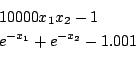Ranges: [-1e8,1e8]

Solving method: HessianSolve+HullConsistency+3B
Solutions:: 2 (exact)
Computation time (April 2003):

 Evo 410C (1.2Ghz) 0.01s

## Collins

Origin: 
Physical meaning: allows to determine the configuration of a parallel robot being given its joint coordinates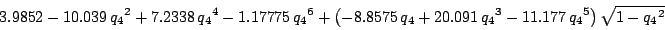Range: [-1,1]

Solving method: GradientSolve,HullConsistency
Solutions:: 6 (exact)
Computation time (April 2003):

 EVO 410C (1.2GHz) 0.2s

## comp.soft-sys.math.maple-14706

Origin: Article 14706 of comp.soft-sys.math.maple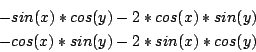Ranges: [0,2Pi]

Solving method: HessianSolve+3B
Solutions:: 13 (exact)
Computation time (November 2005):

 Sun Blade 11.36s

## Design problem

Origin: 
9 equations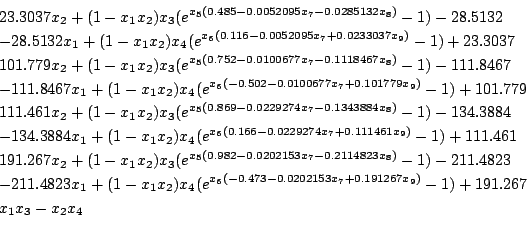23.3037 x_2+(1-x_1 x_2)x_3 (e^{x_5 ( 0.485- 0.0052095 x_7- 0.0285132 x_8)}-1)- 28.5132
- 28.5132 x_1+(1-x_1 x_2)x_4 (e^{x_6 ( 0.116- 0.0052095 x_7+ 0.0233037 x_9)}-1)+ 23.3037
101.779 x_2+(1-x_1 x_2)x_3 (e^{x_5 ( 0.752- 0.0100677 x_7- 0.1118467 x_8)}-1)- 111.8467
- 111.8467 x_1+(1-x_1 x_2)x_4 (e^{x_6 (- 0.502- 0.0100677 x_7+ 0.101779 x_9)}-1)+ 101.779
111.461 x_2+(1-x_1 x_2)x_3 (e^{x_5 ( 0.869- 0.0229274 x_7- 0.1343884 x_8)}-1)- 134.3884
- 134.3884 x_1+(1-x_1 x_2)x_4 (e^{x_6 ( 0.166- 0.0229274 x_7+ 0.111461 x_9)}-1)+ 111.461
191.267 x_2+(1-x_1 x_2)x_3 (e^{x_5 ( 0.982- 0.0202153 x_7-0.2114823 x_8)}-1)- 211.4823
- 211.4823 x_1+(1-x_1 x_2)x_4 (e^{x_6 (- 0.473- 0.0202153 x_7+ 0.191267 x_9)}-1)+ 191.267
x_1 x_3-x_2 x_4

Ranges: [0,10]

Solving method: HessianSolve+HullConsistencyStrong +3B
Solutions:: 1 (exact)
Computation time:

 Cluster (11 PC's) 3h 49mn (April 2003) DELL D400 (1.7GHz) 398s (May 2004)

## DiGregorio

Origin:Digregorio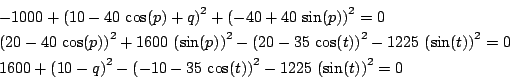Ranges: for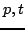[0,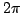], for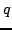[-1000,1000]

Solving method: GradientSolve+3B
Solutions:: 4 (exact)
Computation time (September 2006):

 DELL D400 (1.7Ghz) 0.55s

## Electrical circuit

Origin:Quateroni A, Sacco, R. and Saleri, F., Numerical mathematics, Springer 2000, pp. 413

Solve in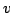: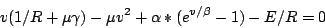with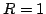,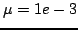,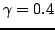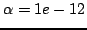,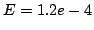,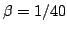Ranges: [-1e8,1e8]
Solving method: HessianSolve+HullConsistencyStrong
Solutions:: 1 (exact)
Computation time:

 DELL D400 (1.7GHz) 0.003s (February 2006)

## Extended Powell

Origin: 
n=2m equations: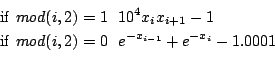Ranges: [-100,100] Solving method: HessianSolve+HullConsistencyStrong+3B
Solutions:: 32 (n=10), 200 (n=20) (exact)
Computation time:

 Evo 410C (1.2Ghz) 23.32s (n=10) (April 2003) DELL D400 (1.7Ghz) 1.72s (n=10), 88.48s (n=20) (May 2004)

## Ferraris

Origin: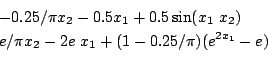-0.25/Pi*x2 - 0.5*x1  + 0.5*sin(x1*x2);
exp(1)/Pi*x2 - 2*exp(1)*x1  + (1 - 0.25/Pi)*(exp(2*x1) -exp(1));

Ranges: [-1e8,1e8]
Solving method: HessianSolve+HullConsistency+3B
Solutions:: 12 (exact)
Computation time (April 2003):
 Evo 410C (1.2Ghz) 0.16s

## Gaussian

Origin:COPRIN, derived from 
3 equations: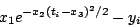with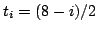, y1=0.0009, y2=0.0044, y3=0.0175

Ranges: [-9,9] for all unknowns

Solving method: HessianSolve+HullConsistencyStrong+3B
Solutions:: 1 (exact)
Computation time:

 Evo 410C (1.2Ghz) 12.84s (April 2003) DELL D400 (1.7GHz) 2.33s (May 2004)

## Geometrica1

Origin:COPRIN, derived from a mesh problem submitted by M. Pouget and F. Cazals from the Geometrica project.
2 equations, 2 unknowns:


(36/25+(x+1)^2)^(1/2)+1/2*(36/25+(1+y)^2)^(1/2)+1/2*(72/25+(-x+1+y)^2)^(1/2)+
(36/25+(1-x)^2)^(1/2)+1/2*(36/25+(2-y)^2)^(1/2)+
1/2*(72/25+(x+2-y)^2)^(1/2)-4*2^(1/2)-3^(1/2)=0

signum(-1/(36/25+(1+y)^2)^(1/2))*arccos((1+y)/(36/25+(1+y)^2)^(1/2))+Pi+
2*signum(-1/(36/25+(1-x)^2)^(1/2))*arccos((1-x)/(36/25+(1-x)^2)^(1/2))+
2^(1/2)*signum(-1/(72/25+(x+2-y)^2)^(1/2))*
arccos((x+2-y)/(72/25+(x+2-y)^2)^(1/2))+
signum(-1/(36/25+(2-y)^2)^(1/2))*arccos((2-y)/(36/25+(2-y)^2)^(1/2))+
((x-1)^2+y^2+36/25)^(1/2)*
signum((366+150*y^2+150*x^2-300*x)/(72/25+(-x+1+y)^2)^(1/2)/(36/25+(1-x)^2)^(1/2))*
arccos(1/25*(61+25*x^2-50*x-25*x*y+25*y)/(72/25+(-x+1+y)^2)^(1/2)/(36/25+(1-x)^2)^(1/2))+
2*signum(-1/(36/25+(x+1)^2)^(1/2))*
arccos((x+1)/(36/25+(x+1)^2)^(1/2))+(x^2+(y-2)^2+36/25)^(1/2)*
signum((816+150*y^2-600*y+150*x^2)/(72/25+(x+2-y)^2)^(1/2)/(36/25+(2-y)^2)^(1/2))*
arccos(1/25*(136-25*x*y-100*y+25*y^2+50*x)/(72/25+(x+2-y)^2)^(1/2)/(36/25+(2-y)^2)^(1/2))+
(x^2+(1+y)^2+36/25)^(1/2)*
signum((366+300*y+150*y^2+150*x^2)/(72/25+(-x+1+y)^2)^(1/2)/(36/25+(1+y)^2)^(1/2))*
arccos(1/25*(61-25*x+50*y-25*x*y+25*y^2)/(72/25+(-x+1+y)^2)^(1/2)/(36/25+(1+y)^2)^(1/2))+
((x+1)^2+(y-1)^2+36/25)^(1/2)*
signum((300*x-300*y+516+150*y^2+150*x^2)/(72/25+(x+2-y)^2)^(1/2)/(36/25+(x+1)^2)^(1/2))*
arccos(1/25*(86+25*x^2+75*x-25*x*y-25*y)/(72/25+(x+2-y)^2)^(1/2)/(36/25+(x+1)^
2)^(1/2))
-4*2^(1/2)*arccos(1/3*3^(1/2)*2^(1/2))+2^(1/2)*
signum(-1/(72/25+(-x+1+y)^2)^(1/2))*
arccos((-x+1+y)/(72/25+(-x+1+y)^2)^(1/2))+((x-1)^2+(y-2)^2+36/25)^(1/2)*
signum((966+150*y^2-600*y+150*x^2-300*x)/(36/25+(1-x)^2)^(1/2)/(36/25+(2-y)^2)^(1/2))*
arccos((x-1)*(y-2)/(36/25+(1-x)^2)^(1/2)/(36/25+(2-y)^2)^(1/2))+((x+1)^2+(1+y)^2+36/25)^(1/2)*
signum((516+300*y+150*y^2+150*x^2+300*x)/(36/25+(x+1)^2)^(1/2)/(36/25+(1+y)^2)^(1/2))*
arccos((x+1)*(1+y)/(36/25+(x+1)^2)^(1/2)/(36/25+(1+y)^2)^(1/2))+
2*2^(1/2)*arccos(1/3*3^(1/2))-2/3*3^(1/2)*Pi =0

where signum(x)=1 if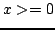, -1 if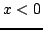Ranges: [-1,1] for all unknowns

Solving method: GeneralSolve
Solutions:: 0 (exact)
Computation time:

 DELL D400 (1.7GHz) 1.7s (July 2004)

## Helical

Origin: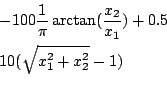Range: x1 in [-1e8,-1e-3], x2 in [-1e8,1e8]

Solving method: GradientSolve,3B
Solutions:: 1 (exact)
Computation time (April 2003):

 EVO 410C (1.2GHz) 0.04s

## Helical1

Origin: COPRIN, derived from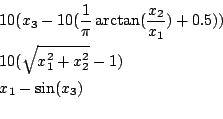Range: x1 in [-1e8,-1e-3], x2,x3 in [-1e8,1e8]

Solving method: GradientSolve,3B
Solutions:: 2 (exact)
Computation time (April 2003):

 EVO 410C (1.2GHz) 0.08s

## Helical valley

Origin: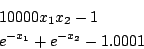Range: x1 in [-1e8,1e8], x2 in [-1e8,1e8]

Solving method: GradientSolve,3B
Solutions:: 1 (exact)
Computation time (April 2003):

 DELL D620 (1.7GHz) 0.02s

## Jennrich1

Origin: COPRIN, derived from 
2 equations with 2 unknowns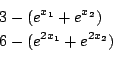Range: [-5,5] for all unknowns

Solving method: HessianSolve,3B
Solutions:: 2 (exact)
Computation time (April 2003):

 EVO 410C (1.2GHz) 0.01s

## Kincox modified

Origin: COPRIN, derived from Kincox COCONUT
2 equations with 2 unknowns with a =1, b=4, l1=10, l2=6


-1.+6.*cos(t1)*cos(t2)-6.*sin(t1)*sin(t2)+10.*cos(t1)=0
-4.+6.*cos(t1)*sin(t2)+6.*cos(t2)*sin(t1)+10.*sin(t1)=0

Ranges: [0,2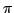] for all unknowns

Solving method: GradientSolve+HullConsistencyStrong+ +3B
Solutions:: 2 (exact)
Computation time (May 2004):

 DELL D400 (1.7GHz) 0.04s

## Kin1 modified

Origin: COPRIN, modified from COCONUT kin1


sin(t2)*cos(t5)*sin(t6)-sin(t3)*cos(t5)*sin(t6)-sin(t4)*cos(t5)*sin(t6)+
cos(t2)*cos(t6)+cos(t3)*cos(t6)+cos(t4)*cos(t6)-0.4077 =0

cos(t1)*cos(t2)*sin(t5)+cos(t1)*cos(t3)*sin(t5)+cos(t1)*cos(t4)*sin(t5)
+sin(t1)*cos(t5)-1.9115 =0

sin(t2)*sin(t5)+sin(t3)*sin(t5)+sin(t4)*sin(t5)-1.9791 =0

cos(t1)*cos(t2)+cos(t1)*cos(t3)+cos(t1)*cos(t4)+cos(t1)*cos(t2)+
cos(t1)*cos(t3)+cos(t1)*cos(t2)-4.0616 =0

sin(t1)*cos(t2)+sin(t1)*cos(t3)+sin(t1)*cos(t4)+sin(t1)*cos(t2)+sin(t1)*cos(t3)+sin(t1)*cos(t2)-1.7172
=0

sin(t2)+sin(t3)+sin(t4)+sin(t2)+sin(t3)+sin(t2)-3.9701 =0

Ranges: [0,]

Solving method: HessianSolve+3B+HullConsistencyStrong
Solutions:: 16 (exact)
Computation time:

 DELL D400 (1.7GHz) 155.18s (August 2004)

## Lambert

Origin: a classical astronomy problem

We define:

r0,r;
11                    10
.138256930224638 10  , .68876844473745 10
mu:=13.271244e10:
dnu:=Pi/4.:
A:=sqrt(r0*r*(1+cos(dnu))):
S:=(u-sin(u))/u^3:
y:=r0+r-A*sin(u)/sqrt(1-cos(u)):
x:=u*sqrt(y)/sqrt(1-cos(u)):

The equation to solve in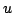is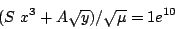Ranges: [0.1, 2-0.1]

Solving method: GradientSolve+3B
Solutions:: 1 (exact)
Computation time:

 DELL D400 (1.7GHz) 0.11s (September 2006)

## Lambert1

Origin: another formulation of a classical astronomy problem

We define:

r0,r;
11                    10
.138256930224638 10  , .68876844473745 10
mu:=13.271244e10:

We then define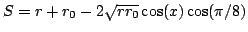. The equation to solve in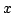is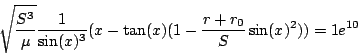Ranges: [0.1,/2-0.1]

Solving method: GradientSolve+3B
Solutions:: 1 (exact)
Computation time:

 DELL D400 (1.7GHz) 0.04s (September 2006)

## Mixed algebraic-trigonometric

Origin:sci.math.num-analysis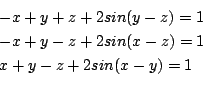Ranges: [-1e8,1e8]

Solving method: HessianSolve+HullConsistency+3B
Solutions:: 1 (exact)
Computation time:

 Sun Blade 6.85s (April 2003) DELL D400 (1.7GHz) 0.1s (May 2004)

## Powell

Origin: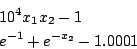Ranges: [-100,100] for all unknowns

Solving method: HessianSolve+HullConsistency+3B
Solutions:: 2 (exact)
Computation time (April 2003):

 Evo 410C (1.2Ghz) 0.01s

## Puma modified

Origin: COPRIN, modified from COCONUT Puma
Physical meaning: inverse kinematics of a 3R robot, the angles t1,t2,t3 are the angles of the three joints
4 equations with 4 unknowns


.4731e-2*cos(t1)*cos(t2)-.3578*sin(t1)*cos(t2)-.1238*cos(t1)
-.1637e-2*sin(t1)-.9338*sin(t2)+cos(t4)-.3571=0

.2238*cos(t1)*cos(t2)+.7623*sin(t1)*cos(t2)+.2638*cos(t1)
-.7745e-1*sin(t1)-.6734*sin(t2)-.6022=0

sin(t3)*sin(t4)+.3578*cos(t1)+.4731e-2*sin(t1)=0

-.7623*cos(t1)+.2238*sin(t1)+.3461=0

Ranges: [0,] for all unknowns

Solving method: GradientSolve+HullConsistencyStrong+ +3B
Solutions:: 16 (exact)
Computation time (May 2004):

 DELL D400 (1.7GHz) 0.48s
See also the algebraic puma

## Reactor

Origin: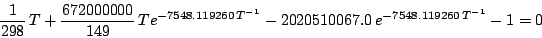Ranges: [100,1000]

Solving method: HessianSolve+HullConsistency+3B
Solutions:: 3 (exact)
Computation time (April 2003):

 Evo 410C (1.2Ghz) 0.06s

## Rump univariate

Origin: mentioned by Rump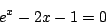Ranges: [-1e8,1e8]

Solving method: HessianSolve,3B
Solutions:: 2 (exact)
Computation time (September 2006):

 DELL D400 (1.7GHz) 0.006s

## sci.math.num-analysis1

Origin: Article 86825 of sci.math.num-analysis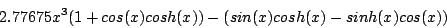Ranges: [-100,100]

Solving method: HessianSolve+HullConsistency+3B
Solutions:: 65 (49 approximate, 16 exact)
Computation time (September 2005):

 DELL D400 (1.2Ghz) 0.06s
Solving method: Maple, based on intpakX
Solutions:: 65 (1 approximate, 64 exact)
Computation time (November 2005):
 DELL D400 (1.2Ghz) 1069s

## sci.math.num-analysis-89741

Origin: Article 89741 of sci.math.num-analysis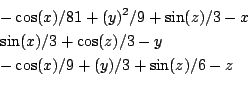Ranges: [-1e8,1e8] for all unknowns (may be largely improved..)

Solving method: HessianSolve+HullConsistency+3B
Solutions:: 1, exact
Computation time (April 2006):

 DELL D400 (1.2Ghz)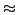0.008s

## sci.math.num-analysis90897

Origin: Article 90897 of sci.math.num-analysis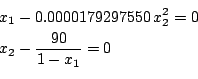Ranges: [-1000,0.99],[-1e8,1e8]

Solving method: HessianSolve+3B
Solutions:: 2 (exact)
Computation time (June 2006):

 Sun Blade 0.13s

## sci.math.num-analysis92191

Origin: Article 92191 of sci.math.num-analysis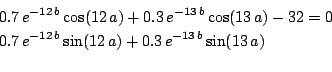Ranges: [0,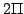],[-10,30]

Solving method: HessianSolve or GradientSolve+3B
Solutions:: 14 (exact)
Computation time (September 2006):

 DELL D400 (1.7GHz) 21.3s (GradientSolve), 68.15s (HessianSolve)

## sci.math.num-analysis97384

Origin: Article 97384 of sci.math.num-analysis
Modified by COPRIN to scale the unknown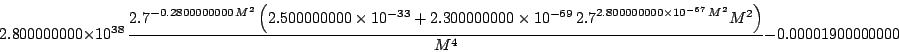Ranges: [1e-4,1000]

Solving method: HessianSolve+3B
Solutions:: 1 (exact)
Computation time (July 2007):

 DELL D620 (1.7Ghz) 0.01s

## Semi-conductor (Molenaar)

Origin: J. Molenaar, P.W. Hemker: A multigrid approach for the solution of the 2D semiconductor equations. IMPACT Comput. Sci. Eng. 2, No. 3, p. 219-243 (1990).
6 equations with a=38.683, ni=1.22e10, V=100, DDD=10.e17.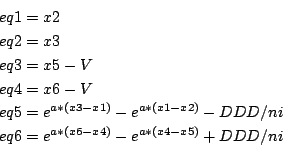Ranges: [-400,400]

Solving method: HessianSolve+HullConsistency+3B
Solutions:: 1 (exact)
Computation time (April 2003):

 Sun Blade 0.16s

## Sinxx

Origin: COPRIN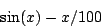Range: [0,A], where A may be chosen arbitrary large without any change in the computation time

Solving method: GradientSolve,HullConsistency
Solutions:: 32 (exact)
Computation time (April 2003):

 Sun Blade 0.051s

## Sinxx1

Origin: COPRIN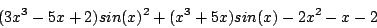Range: [-10,10]

Solving method: GradientSolve,HullConsistency
Solutions:: 9 (exact)
Computation time (April 2003):

 Sun Blade 0.062s

## Sjirk-Boon

Origin: news from Sjirk-Boon, modified by COPRIN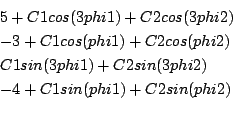Range: [-100,100] for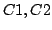,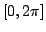for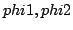Solving method: GradientSolve,Simp2B, 3B
Solutions:: 8 (exact)
Computation time:

 Dell D620 (1.7GHz) (May 2007) 94s

## stoutemyer-eq-2007

Origin: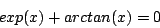Range: [-1e8,1e8]

Solving method: GradientSolve
Solutions:: 1 (exact)
Computation time (November 2007):

 DELL D620 0.01s

## Trigexp1

Origin: 
n equations: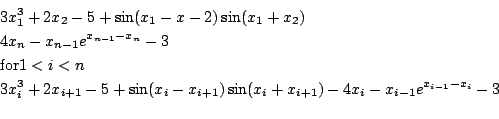for n=20
Ranges: [-100,100]

Solving method: HessianSolve+HullConsistencyStrong+3B
Solutions:: 1 (exact) for n=20,30, 50
Computation time:

 Evo 410C (1.2Ghz) 693.88s (April 2003) DELL D400 (1.7GHz) 0.9s (n=20), 2.3s (n=30) 12.9s (n=50) (May 2004)

## Trigexp2

Origin: 
n=2m+1 equations: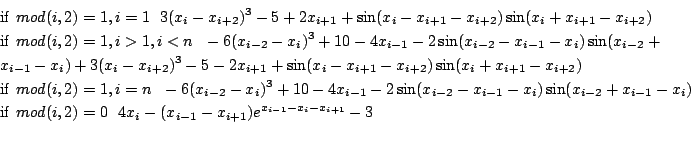Ranges: [-200,200]

Solving method: HessianSolve or GradientSolve +HullConsistencyStrong+ 3B
Solutions:: 0 (n=5,7,9,11) (exact)
Computation time (May 2004):

 DELL D400 (1.7Ghz) 15.31s (n=5) 58.59s (n=7) 115.3s (n=9) 327s (n=11)
This system may be simplified by introducing the new variables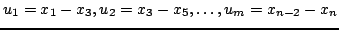i.e. m new variables and the corresponding new equations. We end up with a system of m+n equations in the m+n unknowns xi, ui.

Solutions:: 0 (n=21) (exact)
Computation time (April 2003):

 Evo 410C (1.2Ghz) 2202s

## Trigexp3

Origin: 
n equations with h=1/(n+1):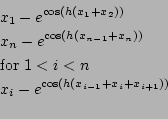for n=20,40
Ranges: [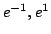]

Solving method: HessianSolve+HullConsistency+3B
Solutions:: 1 for n=20, 40 (exact)
Computation time (April 2003):

 Evo 410C (1.2Ghz) 0.51s (n=20), 2.64s (n=40)

## Trigo1

Origin: 
n equations: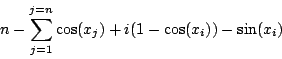for n=5
Ranges: [0.1,-0.1]

Solving method: HessianSolve+3B+HullConsistencyStrong
Solutions:: 1 (exact)
Computation time:

 Evo 410C (1.2GHz) 1.09s (April 2003) DELL D400 (1.7GHz) 0.36s (May 2004)

## Troesch

Origin: 
n equations with h=1/(n+1),R =10: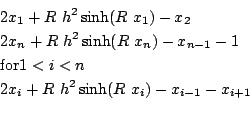for n=10,20
Ranges: [-10,10]

Solving method: HessianSolve+HullConsistency+3B
Solutions:: 1 for n=10, 15 (exact)
Computation time (April 2003):

 Evo 410C (1.2GHz) 32.83s (n=10), 407s (n=15)

## Xu

Origin: 
2 equations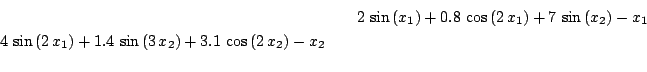Ranges: [-20,20] for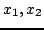Solving method: GradientSolve+Simp2B+3B
Solutions:: 29 (exact)
Computation time:

 DELL D620 (1.7GHz), (July 2007) 0.037s

## Zufiris

Origin: 
4 equations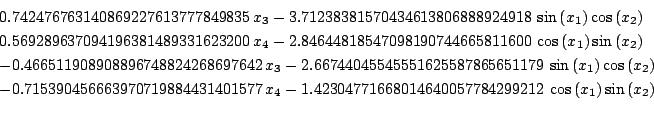Ranges: [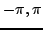] for, [-1.5,1.5] for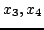Solving method: HessianSolve+Simp2B+3B
Solutions:: 13 (exact)
Computation time:

 DELL D620 (1.7GHz), (July 2007) 0.07sNext: Optimization Up: benches Previous: Polynomial systems
• La page de J-P. Merlet
• J-P. Merlet home page
• La page Présentation de COPRIN
• COPRIN home page
• La page "Présentation" de l'INRIA
• INRIA home page

Jean-Pierre Merlet